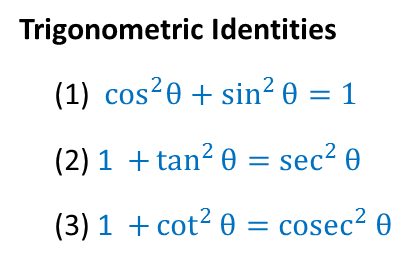Learn all Concepts of Chapter 8 Class 10 (with VIDEOS). Check - Trigonometry - Class 10

Trigonometric Identities are

1. cos 2  θ + sin 2  θ = 1
2. 1 + tan 2  θ = sec 2  θ
3. 1 + cot 2  θ = cosec 2  θ## How do we remember them?

We should only remember

cos 2  θ + sin 2  θ = 1

For other identities,

just divide by sin 2  θ or cos 2  θ

cos 2  θ + sin 2  θ = 1

Divide by  cos 2  θ

cos 2  θ/cos 2  θ + sin 2  θ/cos 2  θ = 1/cos 2  θ

1 + tan 2  θ = sec 2  θ

cos 2  θ + sin 2  θ = 1

Divide by  sin 2  θ

cos 2  θ/sin 2  θ + sin 2  θ/sin 2  θ = 1/cosec 2  θ

cot 2  θ + 1 = cosec 2  θ

1. Chapter 8 Class 10 Introduction to Trignometry
2. Concept wise
3. Evaluating using Trignometric Identities

Evaluating using Trignometric Identities